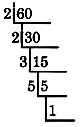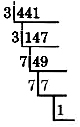# 1.2 Prime factorization

 Page 1 / 1
This module is from Elementary Algebra by Denny Burzynski and Wade Ellis, Jr. This chapter contains many examples of arithmetic techniques that are used directly or indirectly in algebra. Since the chapter is intended as a review, the problem-solving techniques are presented without being developed. Therefore, no work space is provided, nor does the chapter contain all of the pedagogical features of the text. As a review, this chapter can be assigned at the discretion of the instructor and can also be a valuable reference tool for the student.

## Overview

• Prime And Composite Numbers
• The Fundamental Principle Of Arithmetic
• The Prime Factorization Of A Whole Number

## Prime and composite numbers

Notice that the only factors of 7 are 1 and 7 itself, and that the only factors of 23 are 1 and 23 itself.

## Prime number

A whole number greater than 1 whose only whole number factors are itself and 1 is called a prime number.

The first seven prime numbers are

2, 3, 5, 7, 11, 13, and 17

The number 1 is not considered to be a prime number, and the number 2 is the first and only even prime number.
Many numbers have factors other than themselves and 1. For example, the factors of 28 are 1, 2, 4, 7, 14, and 28 (since each of these whole numbers and only these whole numbers divide into 28 without a remainder).

## Composite numbers

A whole number that is composed of factors other than itself and 1 is called a composite number. Composite numbers are not prime numbers.

Some composite numbers are 4, 6, 8, 10, 12, and 15.

## The fundamental principle of arithmetic

Prime numbers are very important in the study of mathematics. We will use them soon in our study of fractions. We will now, however, be introduced to an important mathematical principle.

## The fundamental principle of arithmetic

Except for the order of the factors, every whole number, other than 1, can be factored in one and only one way as a product of prime numbers.

## Prime factorization

When a number is factored so that all its factors are prime numbers, the factorization is called the prime factorization of the number.

## Sample set a

Find the prime factorization of 10.

$10=2\text{\hspace{0.17em}}·\text{\hspace{0.17em}}5$

Both 2 and 5 are prime numbers. Thus, $2\text{\hspace{0.17em}}·\text{\hspace{0.17em}}5$ is the prime factorization of 10.

Find the prime factorization of 60.

$\begin{array}{lllll}60\hfill & =\hfill & 2\text{\hspace{0.17em}}·\text{\hspace{0.17em}}30\hfill & \hfill & \text{30\hspace{0.17em}is\hspace{0.17em}not\hspace{0.17em}prime}.\text{\hspace{0.17em}}30=2\text{\hspace{0.17em}}·\text{\hspace{0.17em}}15\hfill \\ \hfill & =\hfill & 2\text{\hspace{0.17em}}·\text{\hspace{0.17em}}2\text{\hspace{0.17em}}·\text{\hspace{0.17em}}15\hfill & \hfill & 15\text{\hspace{0.17em}is\hspace{0.17em}not\hspace{0.17em}prime}.\text{\hspace{0.17em}}15=3\text{\hspace{0.17em}}·\text{\hspace{0.17em}}5\hfill \\ \hfill & =\hfill & 2\text{\hspace{0.17em}}·\text{\hspace{0.17em}}2\text{\hspace{0.17em}}·\text{\hspace{0.17em}}3\text{\hspace{0.17em}}·\text{\hspace{0.17em}}5\hfill & \hfill & \text{We'll\hspace{0.17em}use\hspace{0.17em}exponents}\text{.\hspace{0.17em}}2\text{\hspace{0.17em}}·\text{\hspace{0.17em}}2={2}^{2}\text{\hspace{0.17em}}\hfill \\ \hfill & =\hfill & {2}^{2}\text{\hspace{0.17em}}·\text{\hspace{0.17em}}3\text{\hspace{0.17em}}·\text{\hspace{0.17em}}5\hfill & \hfill & \hfill \end{array}$

The numbers 2, 3, and 5 are all primes. Thus, $2^{2}·3·5$ is the prime factorization of 60.

Find the prime factorization of 11.

11 is a prime number. Prime factorization applies only to composite numbers.

## The prime factorization of a whole number

The following method provides a way of finding the prime factorization of a whole number. The examples that follow will use the method and make it more clear.

1. Divide the number repeatedly by the smallest prime number that will divide into the number without a remainder.
2. When the prime number used in step 1 no longer divides into the given number without a remainder, repeat the process with the next largest prime number.
3. Continue this process until the quotient is 1.
4. The prime factorization of the given number is the product of all these prime divisors.

## Sample set b

Find the prime factorization of 60.

Since 60 is an even number, it is divisible by 2. We will repeatedly divide by 2 until we no longer can (when we start getting a remainder). We shall divide in the following way.$\begin{array}{l}\text{30\hspace{0.17em}is\hspace{0.17em}divisible\hspace{0.17em}by\hspace{0.17em}2\hspace{0.17em}again}\text{.}\hfill \\ \text{15\hspace{0.17em}is\hspace{0.17em}not\hspace{0.17em}divisible\hspace{0.17em}by\hspace{0.17em}2,\hspace{0.17em}but\hspace{0.17em}is\hspace{0.17em}divisible\hspace{0.17em}by\hspace{0.17em}3,\hspace{0.17em}the\hspace{0.17em}next\hspace{0.17em}largest\hspace{0.17em}prime}\text{.}\hfill \\ \text{5\hspace{0.17em}is\hspace{0.17em}not\hspace{0.17em}divisible\hspace{0.17em}by\hspace{0.17em}3,\hspace{0.17em}but\hspace{0.17em}is\hspace{0.17em}divisible\hspace{0.17em}by\hspace{0.17em}5,\hspace{0.17em}the\hspace{0.17em}next\hspace{0.17em}largest\hspace{0.17em}prime}\text{.}\hfill \\ \text{The\hspace{0.17em}quotient\hspace{0.17em}is\hspace{0.17em}1\hspace{0.17em}so\hspace{0.17em}we\hspace{0.17em}stop\hspace{0.17em}the\hspace{0.17em}division\hspace{0.17em}process}\text{.}\hfill \end{array}$

The prime factorization of 60 is the product of all these divisors.

$\begin{array}{lllll}60\hfill & =\hfill & 2\text{\hspace{0.17em}}·\text{\hspace{0.17em}}2\text{\hspace{0.17em}}·\text{\hspace{0.17em}}3\text{\hspace{0.17em}}·\text{\hspace{0.17em}}5\hfill & \hfill & \text{We\hspace{0.17em}will\hspace{0.17em}use\hspace{0.17em}exponents\hspace{0.17em}when\hspace{0.17em}possible}.\hfill \\ 60\hfill & =\hfill & {2}^{2}\text{\hspace{0.17em}}·\text{\hspace{0.17em}}3\text{\hspace{0.17em}}·\text{\hspace{0.17em}}5\hfill & \hfill & \hfill \end{array}$

Find the prime factorization of 441.

Since 441 is an odd number, it is not divisible by 2. We’ll try 3, the next largest prime.$\begin{array}{l}\text{147\hspace{0.17em}is\hspace{0.17em}divisible\hspace{0.17em}by\hspace{0.17em}3}.\hfill \\ \text{49\hspace{0.17em}is\hspace{0.17em}not\hspace{0.17em}divisible\hspace{0.17em}by\hspace{0.17em}3\hspace{0.17em}nor\hspace{0.17em}by\hspace{0.17em}5},\text{\hspace{0.17em}but\hspace{0.17em}by\hspace{0.17em}7}.\hfill \\ \text{7\hspace{0.17em}is\hspace{0.17em}divisible\hspace{0.17em}by\hspace{0.17em}7}\text{.}\hfill \\ \text{The\hspace{0.17em}quotient\hspace{0.17em}is\hspace{0.17em}1\hspace{0.17em}so\hspace{0.17em}we\hspace{0.17em}stop\hspace{0.17em}the\hspace{0.17em}division\hspace{0.17em}process}.\hfill \end{array}$

The prime factorization of 441 is the product of all the divisors.

$\begin{array}{lllll}441\hfill & =\hfill & 3\text{\hspace{0.17em}}·\text{\hspace{0.17em}}3\text{\hspace{0.17em}}·\text{\hspace{0.17em}}7\text{\hspace{0.17em}}·\text{\hspace{0.17em}}7\hfill & \hfill & \text{We\hspace{0.17em}will\hspace{0.17em}use\hspace{0.17em}exponents\hspace{0.17em}when\hspace{0.17em}possible}.\hfill \\ 441\hfill & =\hfill & {3}^{2}\text{\hspace{0.17em}}·\text{\hspace{0.17em}}{7}^{2}\hfill & \hfill & \hfill \end{array}$

## Exercises

For the following problems, determine which whole numbers are prime and which are composite.

23

prime

25

27

composite

2

3

prime

5

7

prime

9

11

prime

34

55

composite

63

1044

composite

339

209

composite

For the following problems, find the prime factorization of each whole number. Use exponents on repeated factors.

26

38

$2\text{\hspace{0.17em}}·\text{\hspace{0.17em}}19$

54

62

$2\text{\hspace{0.17em}}·\text{\hspace{0.17em}}31$

56

176

${2}^{4}\text{\hspace{0.17em}}·\text{\hspace{0.17em}}11$

480

819

${3}^{2}\text{\hspace{0.17em}}·\text{\hspace{0.17em}}7\text{\hspace{0.17em}}·\text{\hspace{0.17em}}13$

2025

148,225

${5}^{2}\text{\hspace{0.17em}}·\text{\hspace{0.17em}}{7}^{2}\text{\hspace{0.17em}}·\text{\hspace{0.17em}}{11}^{2}$

how can chip be made from sand
are nano particles real
yeah
Joseph
Hello, if I study Physics teacher in bachelor, can I study Nanotechnology in master?
no can't
Lohitha
where we get a research paper on Nano chemistry....?
nanopartical of organic/inorganic / physical chemistry , pdf / thesis / review
Ali
what are the products of Nano chemistry?
There are lots of products of nano chemistry... Like nano coatings.....carbon fiber.. And lots of others..
learn
Even nanotechnology is pretty much all about chemistry... Its the chemistry on quantum or atomic level
learn
da
no nanotechnology is also a part of physics and maths it requires angle formulas and some pressure regarding concepts
Bhagvanji
hey
Giriraj
Preparation and Applications of Nanomaterial for Drug Delivery
revolt
da
Application of nanotechnology in medicine
has a lot of application modern world
Kamaluddeen
yes
narayan
what is variations in raman spectra for nanomaterials
ya I also want to know the raman spectra
Bhagvanji
I only see partial conversation and what's the question here!
what about nanotechnology for water purification
please someone correct me if I'm wrong but I think one can use nanoparticles, specially silver nanoparticles for water treatment.
Damian
yes that's correct
Professor
I think
Professor
Nasa has use it in the 60's, copper as water purification in the moon travel.
Alexandre
nanocopper obvius
Alexandre
what is the stm
is there industrial application of fullrenes. What is the method to prepare fullrene on large scale.?
Rafiq
industrial application...? mmm I think on the medical side as drug carrier, but you should go deeper on your research, I may be wrong
Damian
How we are making nano material?
what is a peer
What is meant by 'nano scale'?
What is STMs full form?
LITNING
scanning tunneling microscope
Sahil
how nano science is used for hydrophobicity
Santosh
Do u think that Graphene and Fullrene fiber can be used to make Air Plane body structure the lightest and strongest. Rafiq
Rafiq
what is differents between GO and RGO?
Mahi
what is simplest way to understand the applications of nano robots used to detect the cancer affected cell of human body.? How this robot is carried to required site of body cell.? what will be the carrier material and how can be detected that correct delivery of drug is done Rafiq
Rafiq
if virus is killing to make ARTIFICIAL DNA OF GRAPHENE FOR KILLED THE VIRUS .THIS IS OUR ASSUMPTION
Anam
analytical skills graphene is prepared to kill any type viruses .
Anam
Any one who tell me about Preparation and application of Nanomaterial for drug Delivery
Hafiz
what is Nano technology ?
write examples of Nano molecule?
Bob
The nanotechnology is as new science, to scale nanometric
brayan
nanotechnology is the study, desing, synthesis, manipulation and application of materials and functional systems through control of matter at nanoscale
Damian
how did you get the value of 2000N.What calculations are needed to arrive at it
Privacy Information Security Software Version 1.1a
Good
Please keep in mind that it's not allowed to promote any social groups (whatsapp, facebook, etc...), exchange phone numbers, email addresses or ask for personal information on QuizOver's platform.

#### Get Jobilize Job Search Mobile App in your pocket Now!ByBy OpenStaxBy David GeltnerBy OpenStaxBy RhodesBy MldelatteByBy OpenStaxBy Brooke DelaneyBy Briana HamiltonBy Janet Forrester